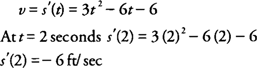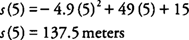## Distance, Velocity, and Acceleration

As previously mentioned, the derivative of a function representing the position of a particle along a line at time t is the instantaneous velocity at that time. The derivative of the velocity, which is the second derivative of the position function, represents the instantaneous acceleration of the particle at time t.

If y = s(t) represents the position function, then v = s′(t) represents the instantaneous velocity, and a = v'(t) = s″(t) represents the instantaneous acceleration of the particle at time t.

A positive velocity indicates that the position is increasing as time increases, while a negative velocity indicates that the position is decreasing with respect to time. If the distance remains constant, then the velocity will be zero on such an interval of time. Likewise, a positive acceleration implies that the velocity is increasing with respect to time, and a negative acceleration implies that the velocity is decreasing with respect to time. If the velocity remains constant on an interval of time, then the acceleration will be zero on the interval.

Example 1: The position of a particle on a line is given by s(t) = t 3 − 3 t 2 − 6 t + 5, where t is measured in seconds and s is measured in feet. Find

a. The velocity of the particle at the end of 2 seconds.

b. The acceleration of the particle at the end of 2 seconds.

Part (a): The velocity of the particle isPart (b): The acceleration of the particle isExample 2: The formula s(t) = −4.9 t 2 + 49 t + 15 gives the height in meters of an object after it is thrown vertically upward from a point 15 meters above the ground at a velocity of 49 m/sec. How high above the ground will the object reach?

The velocity of the object will be zero at its highest point above the ground. That is, v = s′(t) = 0, whereThe height above the ground at 5 seconds ishence, the object will reach its highest point at 137.5 m above the ground.